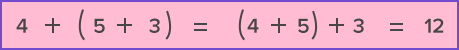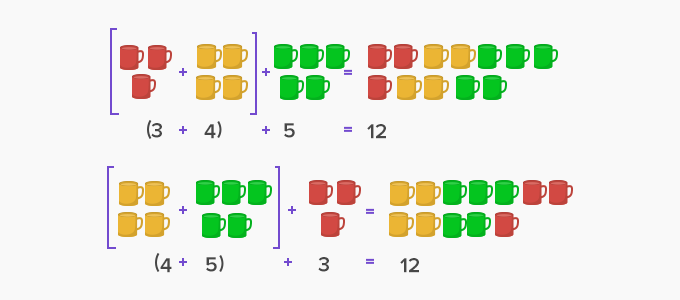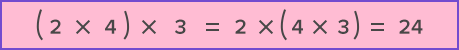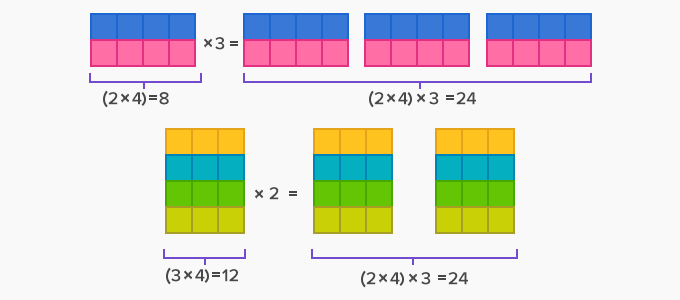# Associative Property - Definition with Examples

The Complete K-5 Math Learning Program Built for Your Child

• 40 Million Kids

Loved by kids and parent worldwide

• 50,000 Schools

Trusted by teachers across schools

• Comprehensive Curriculum

Aligned to Common Core

## What is Associative Property?

This property states that when three or more numbers are added (or multiplied), the sum (or the product) is the same regardless of the grouping of the addends (or the multiplicands).

• Grouping means the use of parentheses or brackets to group numbers.

• Associative property involves 3 or more numbers.

• The numbers that are grouped within a parenthesis or bracket become one unit.

• Associative property can only be used with addition and multiplication and not with subtraction or division.

Example of Associative Property for AdditionExamples of Associative Property for Multiplication:The above examples indicate that changing the grouping doesn't make any changes to the answer.

The associative property is helpful while adding or multiplying multiple numbers. By grouping, we can create smaller components to solve. It makes the calculations of addition or multiplication of multiple numbers easier and faster.

17 + 5 + 3 = (17 + 3) + 5

= 20 + 5

= 25

Here, adding 17 and 3 gives 20. Then, adding 5 to 20 gives 25. The grouping helped to find the answer easily and quickly.

Example Multiplication:

3 × 4 × 25 = (25 × 4) × 3

= 100 × 3

= 300

Here, multiplying 25 by 4 gives 100. Then, 3 can be easily multiplied by 100 to get 300.

However, we cannot apply the associative property to subtraction or division. When we change the grouping of numbers in subtraction or division, it changes the answer and hence, this property is not applicable.

Example Subtraction:

10 – (5 – 2) = 10 - 3 = 7

(10 – 5) – 2 = 5 – 2 = 3

So, 10 – (5 – 2) ≠ (10 – 5) – 2

Example Division:

(24 ÷ 4) ÷ 2 = 6 ÷ 2 = 3

24 ÷ (4 ÷ 2) = 24 ÷ 2 = 12

So, (24 ÷ 4) ÷ 2 ≠ 24 ÷ (4 ÷ 2)

 Fun Facts Associative property gets its name from the word “Associate” and it refers to grouping of numbers.

Won Numerous Awards & Honors